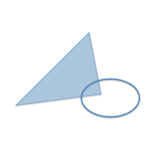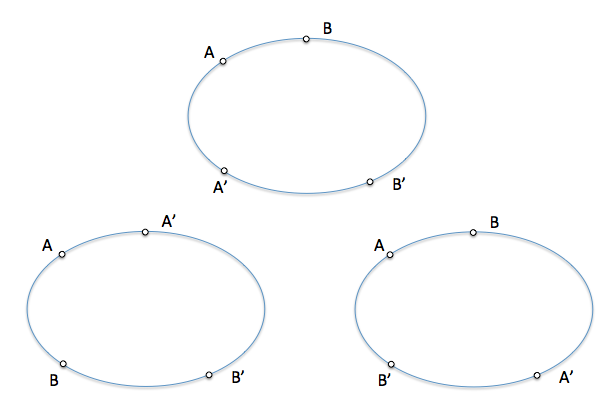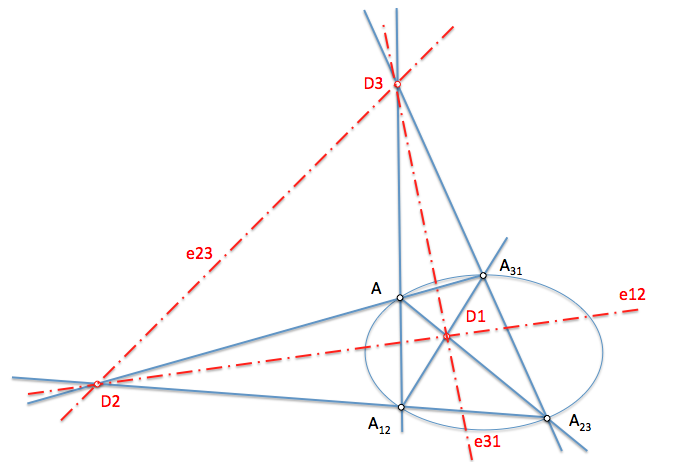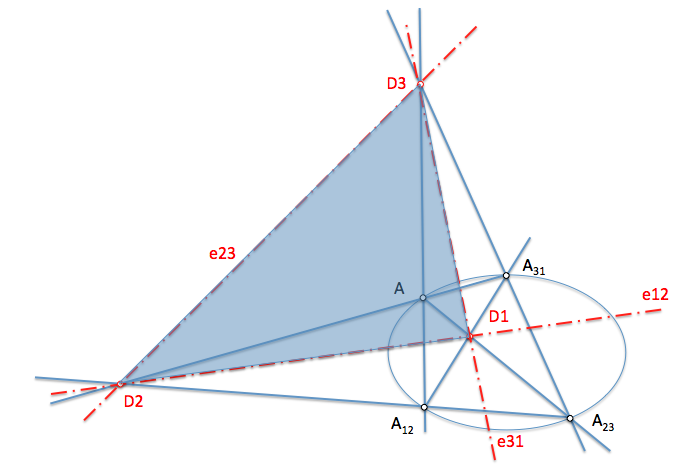# Projective Geometry: Autopolares triangles in Involutions in second-order seriesBy linking four points of a conical proyectivamente by Involutions determine the axis of involution of these proyectividades.

Given the four points needed to define an involution, We can ask ourselves How many different Involutions We can establish between them.

If we call “A” one of the points, the counterpart of this item in a particular involution can be any of the other three, being the pair of points remaining counterparts between if. We can therefore see that three different Involutions are possible as shown in Figure.In each of these Involutions a different involution axis shall be determined.

If we get the three axes of involution on a same figure, We can obtain interesting conclusions.

• If we associate as counterparts points A-A12 We will have like involution to the straight shaft E12
• If we associate as counterparts points A-A23 We will have like the straight shaft e23
• If we associate as counterparts points A-A31 We will have like the straight shaft E31

We see that the three axes of involution coincide with the diagonals of the full cuadrivertice determined by the homologous points of the Conic, so the polar point diagonal with respect to two of the sides of the cuadrivertice is the opposite diagonal (It contains no), as we saw when defining the Polar of a point with respect to two lines.We see that in the triangle determined by three diagonal dots, D1, D2 and D3, each of these points is polar opposite straight. We say that this triangle is “Autopolar” with respect to the given Conic.